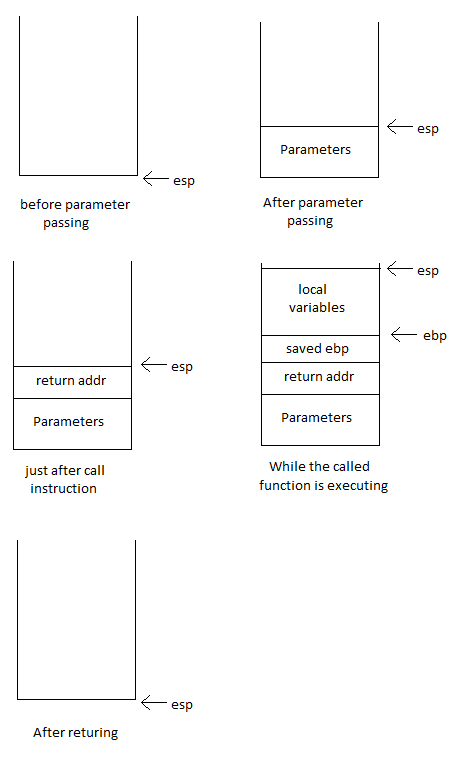# Parameter Passing

The parameters are pushed on the stack and then function calls are made. So the parameters are just below the return address. If a function wants to read the parameters, then it can read from the stack just below the return address.
Let's see this in an example

C code:
```void fun(int x, int y)
{
x++;
x += y;
}
int main()
{
fun(2, 3);
}
```
Here is the generated assembly code:
```.text
.globl fun
fun:
pushl	%ebp
movl	%esp, %ebp
movl	12(%ebp), %eax
popl	%ebp
ret
.globl main
main:
pushl   %ebp
movl    %esp, %ebp
subl    \$8, %esp
movl    \$3, 4(%esp)
movl    \$2, (%esp)
call    fun
leave
ret
```
Location of local variables of the stack (local variables are explained here)
```x ==> 8(%ebp)
y ==> 12(%ebp), %eax
```

This is very much related to what is described in the local variable chapters. The parameters are allocated as the local variables only. The only one difference is that the local variables are allocated on the top where the return address is pushed on the stack and the parameters lie beneath the return address.

The parameter passing can be explained with this diagram:```# .text segment starts
.text

# export the the function fun
.globl fun

# function fun starts here
fun:

# save ebp on stack
pushl   %ebp
movl    %esp, %ebp

# x++;

# tmp = y
movl    12(%ebp), %eax

# x = x + tmp

#restore ebp and return
popl    %ebp
ret

# export the main function
.globl main

# main function starts here
main:
# save the ebp register
pushl   %ebp
movl    %esp, %ebp

# stack_pointer -= 8
subl	\$8, %esp

# push the second parameter
movl    \$3, 4(%esp)

# push the first parameter
movl    \$2, (%esp)

# call the function fun
call	fun

# return the main function
leave
ret
```

Points to understand:
• The parameters are passed left to right. The last parameter in C code is pushed first and the first parameter is pushed in the last. So if you scan the stack from top, then the first parameter will be found first.
• The generated code does not use the push instruction. Instead it decrements the stack pointer first then add parameters to the space created by decrementing the stack pointer. This has been possibly done for perf reasons (subl + movl could be faster than push + pop).
• If the parameter is of type structure then the whole of the structure will be pushed on the stack.

Do you collaborate using whiteboard? Please try Lekh Board - An Intelligent Collaborate Whiteboard App